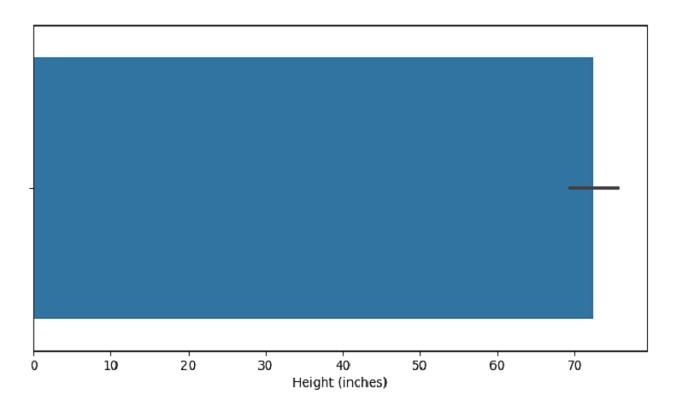# Create a Bar plot with SeaBorn â€“ Python Pandas

Bar Plot in Seaborn is used to show point estimates and confidence intervals as rectangular bars. The seaborn.barplot() is used for this.

Let’s say the following is our dataset in the form of a CSV file − Cricketers.csv

At first, import the required 3 libraries −

import seaborn as sb
import pandas as pd
import matplotlib.pyplot as plt

Load data from a CSV file into a Pandas DataFrame −

dataFrame = pd.read_csv("C:\Users\amit_\Desktop\Cricketers.csv")


Plotting bar plot with Height column −

sb.barplot(x =dataFrame["Height"])

## Example

Following is the code −

import seaborn as sb
import pandas as pd
import matplotlib.pyplot as plt

# Load data from a CSV file into a Pandas DataFrame

# plotting bar plot with Height column
sb.barplot(x =dataFrame["Height"])

# label
plt.xlabel("Height (inches)")

# display
plt.show()

## Output

This will produce the following output −